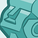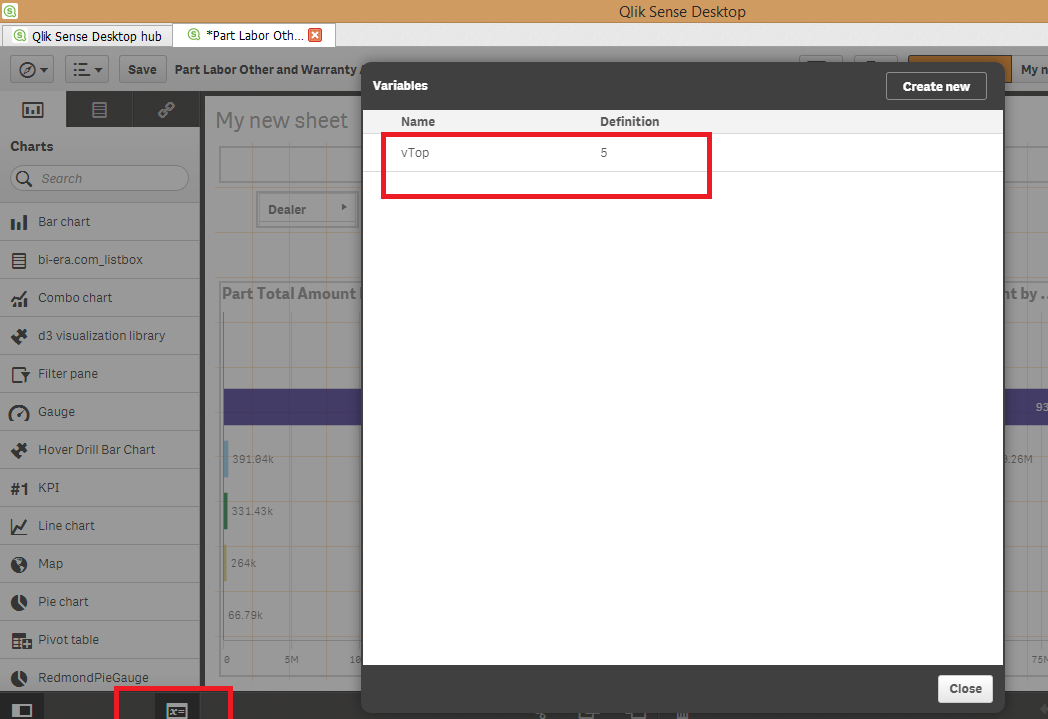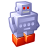# App Development

Announcements
Support Cases coming to Qlik Community Oct. 4! Start chats, open cases, explore resources: READ DETAILS
cancel
Showing results for
Did you mean:Contributor II

## How to make our chart dynamically change based on the customized field Top(5,10,15)

Hi,Here I am creating a new Dimension by giving the expression  \$(vTop)

Here vTop is the variable having the expression  valueList(5,10,15).

Kind Regards.

1 Solution

Accepted SolutionsContributor II
Author

HI,

Here is the solution for this.

1).Download the variable extension from https://github.com/erikwett/qsVariable/raw/master/dist/variable.zip and extract       to extensions folder of Qlik Sense. The variable extension is visible in the charts tab as shown in below image. and         choose Render as field from and give name as vTop save it and use vTop in your above expression for the specific         measure to be used in the chart or table...2).Drag and drop the extension to the work pane.

3).Before that create a variable(here vTop is my variable).4) Give this name reference to the variable extension Field.5)Now write the expression of measure using the variable "vTop" like

Sum({<Dealer = {"=rank(sum(support_count))<= \$(vTop)"}>}support_count)

6)now we can see the Top values based on the entered value.Thanks,

Sattipandu.

6 RepliesPartner

If i am not getting your question wrong you want to limit dimension on the basis of top 5, 10 or 15 measures.

This is what are you asking ?Specialist III

you need to use a key field but this should work

sum({< Key={"=rank(Sum(Value)) < \$(vTop)"}>}Value)Contributor II
Author

YesContributor II
Author

Hi Ramon,

Where can i write this expression, can u please give more clarification on this.

Kind Regards......Specialist III

write this as part of the expression

https://community.qlik.com/docs/DOC-4951

this link will give you some example of set analysisContributor II
Author

HI,

Here is the solution for this.

1).Download the variable extension from https://github.com/erikwett/qsVariable/raw/master/dist/variable.zip and extract       to extensions folder of Qlik Sense. The variable extension is visible in the charts tab as shown in below image. and         choose Render as field from and give name as vTop save it and use vTop in your above expression for the specific         measure to be used in the chart or table...2).Drag and drop the extension to the work pane.

3).Before that create a variable(here vTop is my variable).4) Give this name reference to the variable extension Field.5)Now write the expression of measure using the variable "vTop" like

Sum({<Dealer = {"=rank(sum(support_count))<= \$(vTop)"}>}support_count)

6)now we can see the Top values based on the entered value.Thanks,

Sattipandu.Tags
Community Browser